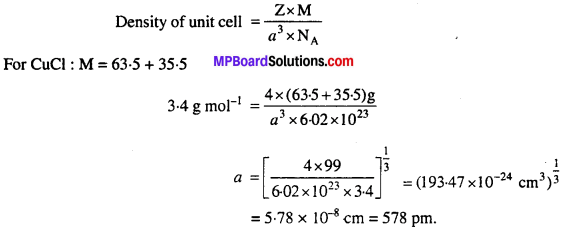# MP Board Class 12th Chemistry Solutions Chapter 1 The Solid State

## MP Board Class 12th Chemistry Solutions Chapter 1 The Solid State

### The Solid State NCERT Intext Exercises

Question 1.
Why are solids rigid?
Solids are rigid due to the presence of strong intermolecular forces between the constituent particles.

Question 2.
Why do solids have a definite volume
In solids, the constituent particles are bonded together by strong attractive forces between them. By the increase or decrease of pressure, the intermolecular space remains unaffected. Thus, the volume of solids is definite.

Question 3.
Classify the following as amorphous or crystalline solids:
Polyurethane, naphthalene, benzoic acid, Teflon, potassium nitrate, cellophane, polyvinyl chloride, fiberglass, copper.
Amorphous solids: Polyurethane, teflon, cellophane, polyvinyl chloride, fibre glass.
Crystalline solids : Benzoic acid, potassium nitrate, copper.

Question 4.
Why is glass considered a supercooled liquid?
Like liquids, glass has tendency to flow but very slowly. Therefore, it is called supercooled liquid.

Question 5.
Refractive index of a solid is observed to have the same value along with all directions. Comment on the nature of this solid. Would it show cleavage property?
Since refractive index of a solid is observed to have the same value along with all directions, the solid must be amorphous. On cutting this, solid will undergo irregular cleavage.

Question 6.
Classify the following solids in different categories based on the nature of intermolecular forces operating in them: Potassium sulphate, tin, benzene, urea, ammonia, water, zinc sulphide, graphite, rubidium, argon, silicon carbide.
Potassium sulphate : Ionic, Tin : Metallic, Benzene : Molecular (non-polar), Urea: Molecular (polar), Ammonia: Molecular (H-bonded), Water: Molecular (H-bonded), Zinc sulphide: Ionic, Graphite: Covalent or network, Rubidium: Metallic, Argon: Molecular (non-polar), Silicon carbide : Covalent or network.

Question 7.
Solid A is a very hard electrical insulator in solid as well as in molten state and melts at extremely high temperature. What type of solid is it ?
Covalent solid.

Question 8.
Ionic solids conduct electricity in molten state but not in solid state. Explain.
In solid-state, the ions are fixed and the ions cannot move in an applied electric field. But in a molten state, the ions are free to move and hence conduct electricity.

Question 9.
What type of solids are electrical conductors, malleable and ductile?
Metallic solids, they are conductor due to the presence of free electron in them.

Question 10.
Give the significance of a ‘lattice point’.
The lattice point represents the position of the constituent particles in the crystal lattice. When lattice points are joined by straight lines, they bring out the geometry of the lattice.

Question 11.
Name the parameters that characterize a unit cell.
A unit cell is characterized by :

1. The dimensions along the three edges. These are represented by a, b, and c.
2. The angle between the edges. These are represented by α,β, and γ. The angle α is between b and c,β is between a and c and γ is between a and b (For diagram refer book).

Question 12.
Distinguish between
1. Hexagonal and monoclinic unit cells
2. Face centred and end centred unit cells.
1. Hexagonal unit cell:
a = b ≠ c and α = β = 90°, γ = 120°
Monoclinic unit cell:
a ≠ b ≠ c and α = γ = 90°, β ≠ 90°

2. Face-centred unit cell:
Particles at the comers as well as at the centre of each face.
Number of particles per unit cell = 8 × $$\frac{1}{8}$$ + 6 × $$\frac{1}{2}$$ = 4

End – centred unit cell:
Particles at the corners and at the centres of two end faces.
Number of particles per unit cell = 8 × $$\frac{1}{8}$$ + 2 × $$\frac{1}{2}$$ = 2

Question 13.
Explain, how many portions of an atom located at (i) corner and (ii) body centre of a cubic unit cell is part of its neighbouring unit cell?

1. In a crystal, each comer of a unit cell is common to 8 unit cells and hence each comer atom is shared by 8 unit cells. Thus, $$\frac{1}{8}$$ atom is part of its neighbouring unit cell.
2. The atom located at the body centre of a cubic unit cell belongs to only one unit cell. Thus it is not shared with the neighbouring unit cell.Question 14.
What is the two-dimensional coordination number of a molecule in a square close-packed layer?
4.

Question 15.
A compound forms a hexagonal close-packed structure. What is the total number of voids in 0-5 mol of it? How many of these are tetrahedral voids?
The number of octahedral voids = N
The number of tetrahedral voids = 2N
∴ Total number of voids = 3N
The number of close packed atoms = 0.5 mol = 0.5 × 6.022 × 1023
∴ Total number of voids = 3 × 0.5 × 6.022 × 1023 = 9.03 × 1023
The number of tetrahedral voids = 2 × 0.5 × 6.022 × 1023 = 6.022 × 1023

Question 16.
A compound is formed by two elements M and N. The element N forms ccp and atoms of M occupy 1/3rd of tetrahedral voids. What is the formula of the compound?
Since N forms ccp arrangement, it will have 4 atoms in a unit cell.
Number of N atoms in unit cell = 4
For each atom, there are two tetrahedral voids so that there are 8 tetrahedral voids per unit cell.
No. of M atoms = $$\frac { 1 }{ 3 }$$ × 8 = $$\frac { 8 }{ 3 }$$
Formula = M8/3 N4 or M2N3.

Question 17.
Which of the following lattices has the highest packing efficiency
(i) simple cubic
(ii) body-centered cubic and
(iii) hexagonal close-packed lattice?
The packing efficiencies are:
Simple cubic = 52.4%
Body centred cubic = 68%
Hexagonal close packed = 74%
∴ Hexagonal close-packed lattice has the highest packing efficiency.

Question 18.
An element with molar mass 2.7 × 10-2 kg mol-1 forms a cubic unit cell with edge length 405 pm. If its density is 2.7 × 103 kg m-3, what is the nature of the cubic unit cell?
We know that Z = $$\frac{a^{3} \times \mathrm{N}_{\mathrm{A}} \times d}{\mathrm{M}}$$
Where a = 405pm = 405 x 10-10
d = 2.7 × 103 kg m-3
M = 2.7 × 10-2 kg m-1
= 2.7g mol-1
NA = 6.203 x 1023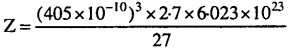Z =  4
∴ The element has fee (cep) unit cell.

Question 19.
What type of defect can arise when a solid is heated? Which physical property is affected by it and in what way?
Vacancy defect. It results in a decrease in the density of the substance since some of the lattice sites are occupied.

Question 20.
What type of stoichiometric defect is shown by : (i) ZnS, (ii) AgBr.
(i) ZnS, shows Frenkel defect due to large difference in size of ions.
(ii) AgBr, shows both Frenkel defect and Schottky defect.

Question 21.
Explain, how vacancies are introduced in an ionic solid when a cation of higher valence is added as an impurity in it ?
When a cation of higher valence is added as an impurity, some of the sites of the original cations are occupied by the cations of higher valence and produce vacant sites.

Question 22.
Ionic solids, which have anionic vacancies due to metal excess defect, develop colour ? Explain with the help of a suitable example.
The anionic vacancies due to metal excess defect in ionic solids are occupied by free electrons to maintain electrical neutrality. These impart colour by excitation of these electrons when they absorb energy from the visible light falling on the crystals. For example :
When NaCl is heated in presence of sodium vapours, Na+ ions are in excess, Cl ions leave their normal site and come to the surface. The vacant site of anion is occupied by electron forming F-centre. They absorb light from visible region and radiate complementary colour.

Question 23.
A group-14 element is to be converted into an n-type semiconductor by doping it with a suitable impurity. To which group should this impurity belong?
n-type semiconductors are obtained by doping of a higher group impurity. Hence, to convert group 14 element to n-type semiconductor, it should be doped with a Group 15 element.

Question 24.
What type of substances would make better permanent magnets, ferromagnetic or ferrimagnetic? Justify your answer.
Ferromagnetic materials would make better permanent magnets than ferrimagnetic materials because, in ferromagnetic solids, the magnetic moments of unpaired electrons spontaneously align themselves in the same direction. However, in ferrimagnetic solids the magnetic moments of the domains are aligned in the parallel and anti-parallel direction in unequal numbers.### The Solid State NCERT Textbook Exercises

Question 1.
Define the term ‘amorphous’. Give a few examples of amorphous solids.
The term ‘amorphous’ (Greek) means ‘no forms’ and in amorphous solids, the constituent particles have only short-range order, e.g. Glass, plastics, rubber.

Question 2.
What makes a glass different from a solid such as quartz? Under what conditions could quartz be converted into glass?
Glass is an amorphous solid in which the constituent particles (SiO4 tetrahedra) have only short-range order and there is no long-range order. On melting quartz and then cooling it rapidly, it is converted into glass.

Question 3.
Classify each of the following solids as ionic, metallic, molecular, network (covalent) or amorphous.
(i) Tetra phosphorus decoxide (P4O10)
(ii) Ammonium phosphate (NH4)3PO4
(iii) SiC
(iv) I2
(v) P4
(vi) Plastic
(vii) Graphite
(viii) Brass
(ix) Rb
(x) LiBr
(xi) Si.
Ionic: Ammonium phosphate, LiBr
Metallic: Brass, Pb
Molecular: I2, P4O10, white phosphorus
Covalent: Graphite, SiC, Si, red phosphorus
Amorphous: Plastic

Question 4.
(i) What is meant by the term ‘coordination number’?
(ii) What is the coordination number of atoms:
(a) In a cubic close-packed structure?
(b) In a body-centered cubic structure?
(i) The coordination number of constituent particles (atom, ion, or molecule) in a crystal is the number of constituent particles which are the immediate neighbor of the particle in the crystal. In ionic crystals, the coordination number of an ion in the crystal is the number of oppositely charged ions surrounding that particular ion.
(ii) a] 12 b] 8.

Question 5.
How can you determine the atomic mass of an unknown metal if you know its density and the dimension of its unit cell? Explain.
Atomic mass (M)Question 6.
‘Stability of a crystal is reflected in the magnitude of its melting points’, comment. Collect melting points of solid water, ethyl alcohol, diethyl ether, and methane from a data book. What can you say about the intermolecular forces between these molecules?
The stability of a crystal depends on the force of attraction so: Higher the melting point of the crystal, the stronger the intermolecular force of attraction, hence greater is the stability of a crystal.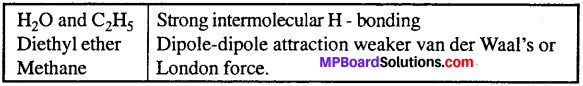Melting point of the H2O, C2H5OH, diethyl ether and methane given as below :On the basis of melting point, the strength of the intermolecular forces between these molecules follows the order :
Water > Diethyl ether > Ethyl alcohol > Methane.

Question 7.
How will you distinguish between the following pairs of terms:
(i) Hexagonal close packing and cubic close packing?
(ii) Crystal lattice and unit cell?
(iii) Tetrahedral void and octahedral void?
(i) During the formation of crystals, the constituent particles are closely packed. In close packing the maximum available space is occupied. The stability of the crystals depends on the extent of close packing. In close packing the solid particles are considered as identical spheres.

(ii) Crystal lattice :
The regular arrangement of the constituent particle (atoms, molecules, or ions) of a crystal in three-dimensional space.

Unit cell :
It is the smallest repeating unit in three-dimensional space, which is repeated again and again to give the complete lattice.

(iii)Tetrahedral void :

1. It is the open space between four touching spheres of two layers of atoms.
2. The radius of tetrahedral void relative to radius of sphere is 0-225.

Octahedral void :

1. It is the open space between six touching spheres of two layers of atoms.
2. The radius of octahedral void relative to radius of sphere is 0-414.

Question 8.
How many lattice points are there in one unit cell of each of the following lattice:
(i) Face centred cubic
(ii) Face centred tetragonal
(iii) Body centred.
Lattice points in face centred cubic or face centred tetragonal = 8 (at corners) + 6 (at face centres) = 14
However, particles per unit cell = 8 × $$\frac{1}{8}+$$ + 6 × $$\frac{1}{2}+$$ =4
lattice points in body centred cube = 8 (at comers) + 1 (at body centre) = 9.
However, particles per unit cell = 8 × $$\frac{1}{8}+$$ + 1 = 2

Question 9.
Explain:
1. The basis of similarities and differences between metallic and ionic crystals.
2. Ionic solids are hard and brittle.

1. Ionic solids:
In ionic solids, the constituent particles are positive and negative ions. The positive ions are surrounded by negative ions and vice versa. The ions are held together by a strong electrostatic force of attraction.

Since the ions are firmly fixed in the crystal lattice, they are hard and brittle with high melting point, high heat of fusion and lattice energy. These are insulators in the solid-state but are good conductors in solution or molten state. e.g. NaCl, MgSO4, CaF2 etc.

2. Molecular solids:
Molecular solids are made up of small discrete molecules. They are subdivided into the following categories.

(a) Non-polar molecular solids:
In these solids, the non-polar molecules like H2, Cl2, I2 etc are held together by weak dispersion forces or London forces. They are soft, non-conductors of electricity and have low melting points.

(b) Polar molecular solids:
Molecules having polar covalent bonds are held together by dipole-dipole interactions. Solid SO2, NH3 etc are examples of this type. They are soft and non-conductors of electricity.

(c) Hydrogen bonded molecular solids:
The molecules are held together by strong hydrogen bonds.
e.g. Ice.

3. Ionic solids have strong interionic attractive forces and hence are hard. When an ionic solid is subjected to distortion, the ions of the same charge are brought close together and the repulsive forces between them cause the crystal to fracture. Hence are brittle.

Question 10.
Calculate the efficiency of packing in the case of a metal crystal for:
(i) Simple cubic
(ii) Body centered cubic
Solution:
(i) Packing efficiency of simple cube(ii) Packing efficiency in body centred cubic structure(iii) Packing efficiency of face centred cubic structureQuestion 11.
Silver crystallizes in fee lattice. If edge length of the cell is 4.077 × 10-8 cm and density is 10-5 gcm-3, calculate the atomic mass of silver.
Solution: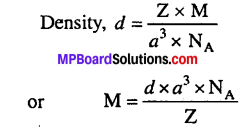Z = 4 (fcc lattice), d = 10.5 gcm, N = 6.022 x 10, (a = 4.077 × 10-8 cm)Question 12.
A cubic solid is made of two elements P and Q. Atoms of Q are at the corners of the cube and P at the body centre. What is the formula of the compound? What are the coordination numbers of P and Q?
As atoms Q are present at the 8 corners of the cube, therefore, number of atoms of in the unit cell = $$\frac { 1 }{ 8 }$$ × 8 = 1
As atoms P are present at the body centre, therefore, number of atoms of x in the unit cell = 1
∴Ratio of atoms x:y =1:1
Hence the formula of the compound is PQ coordination number of each of P and Q = 8.

Question 13.
Niobium crystallizes in a body-centered cubic structure. If density is 8.55 gem-3, calculate atomic radius of niobium using its atomic mass 93u.
Solution:
Density = 8.55 g cm-3
Let, length of the edge = a cm
Number of atoms per unit cell, Z = 2 (bcc)
Atomic mass, M = 93 g mol-1
density, d = $$\frac{\mathrm{Z} \times \mathrm{M}}{a^{3} \times \mathrm{N}_{\mathrm{A}}}$$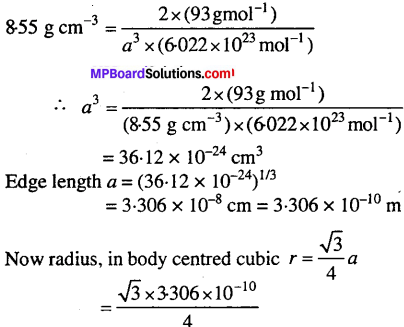= 1.431 x 10-10m = 0.143 nm

Question 14.
If the radius of the octahedral void is r and the radius of the atoms in close packing is R, derive a relation between r and R.
Solution:
Atoms covering the octahedral void from the top and below are not shown in the figure. Centre of the octahedral void is C and its radius is equal to r. Atoms surrounding the voids are of radius R.According to the figure,
Radius of atom surrounding the void BA = R
BC = Radius of void + Radius of outer atom = R + r
∠ABC = 45°
in triangle ABC $$\frac {AB}{BC}$$ = cos 45° = $$\frac{1}{\sqrt{2}}$$ = 0.707
or $$\frac {AB}{BC}$$ = 0.707
or 0.707 R + 0.707 r = R
or o.293 = 0.707r
$$\frac {r}{R}$$ = $$\frac {0.293}{0.707}$$ = 0.414

Question 15.
Copper crystallizes into a fee lattice with edge length 3.61 × 10-8 cm. Show that the calculated density is in agreement with its measured value of 8.92 gcm-3.
Solution:
We know that, d = $$\frac{\mathrm{Z} \times \mathrm{M}}{a^{3} \times \mathrm{N}_{\mathrm{A}}}$$
For fcc Z = 4, Atomic mass of copper = 63.5, a = 3.16 x 10-8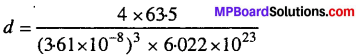= 8.96 g cm-3
This value is close to measured value.Question 16.
The analysis shows that nickel oxide has the formula NiO0.98 O1.00. What fractions of nickel exist as Ni2+ and Ni3+ ions?
Solution:
The formula Ni098 O1.00 shows that Ni : O = 0.98 : 1.00 = 98 : 100.
Thus, if there are 100 O-atoms, then Ni atoms = 98
charge on 100 O2′ ions = 100 x (-2) = – 200
suppose Ni atoms as Ni2+ = x
Then Ni atoms as Ni3+ = 98 – x
The total charge on Ni2+ and Ni3+ = (+2) x + (+3) (98 – x) = 294 – x
As metal oxide is neutral, total charge on
cations = total charge on anions
294 – x = 200
x = 94
∴ % of Ni as Ni2+ = 96%
% of Ni as Ni3+ = 100 – 96 = 4.

Question 17.
What is a semiconductor? Describe the two main types of semiconductors and contrast their conduction mechanism.
The solids which have intermediate conductivities generally between 10-6 to 104-1m-1 are called semiconductors, e.g. Germanium and silicon. The two main types of semiconductors are as follows.

(i) n-type semiconductor:
When a silicon crystal is doped with atoms of group-15 elements, such as P, As, Sb or Bi, then only four of the five valence electrons of each impurity atom participate in forming covalent bonds and fifth electron is almost free to conduct electricity. Silicon that has been doped with a group-15 element is called an n-type semiconductor.

(ii) p-type semiconductor:
When a silicon crystal is doped with atoms of group-13 elements, such as B, Al, Ga or In, each impurity atom forms only three covalent bonds with the host atoms. The place where the fourth electron is missing is called a hole which moves through the crystal-like positive charge and hence increases its conductivity. Silicon that has been doped with group-13 elements is called a p-type semiconductor.

Question 18.
Non-stoichiometric cuprous oxide, Cu2O can be prepared in laboratory. In this oxide, copper to oxygen ratio is slightly less than 2:1. Can you account for the fact that this substance is a p-type semiconductor?
The ratio less than 2:1 in Cu2O shows that some cuprous (Cu+) ions have been replaced by cupric (Cu2+) ions. To maintain electrical neutrality, every two Cu+ ions will be replaced by one Cu2+ ion thereby creating a hole. As conduction will be due to presence of these positive holes, hence it is a p-type semiconductor.

Question 19.
Ferric oxide crystallizes in a hexagonal close-packed array of oxide ions with two out of every three octahedral holes occupied by ferric ions. Derive the formula of the ferric oxide.
Solution:
O2- ions form cubic close-packed lattice.
∴ Number of Fe3+ = $$\frac{2}{3}$$ of octahedral voids = $$\frac{2}{3}$$
∴ Formula of the compound = $$\mathrm{Fe}_{3, \mathrm{O}}^{3+} \mathrm{O}^{2-}$$ = Fe2O3

Question 20.
Classify each of the following as being either a p-type or an-type semiconductor :
(i) Ge doped with In
(ii) B doped with Si.
(i) Ge is group 14 element and In is group 13 element. Hence, an electron deficit hole is created, and therefore, it is p-type.
(ii) B is group 13 element and Si is group 14 element, there will be the free electron. Hence it is p-type.

Question 21.
Gold (atomic radius = 0.144 nm) crystallizes in a face-centered unit cell. What is the length of a side of the cell?
Solution:
For fee lattice, edge length, a = 2$$\sqrt{2}$$r = 2$$\sqrt{2}$$ × 0.144 nm = 0.407 nm

Question 22.
In terms of band theory, what is the difference:
(i) between a conductor and an insulator?
(ii) between a conductor and a semiconductor?
(i) The energy gap between the valence band and the conduction band in an insulator is very large whereas in a conductor the energy gap is either very small or there is overlapping between the valence band and conduction band.

(ii) In a conductor, the energy gap between the valence band and conduction band is very small or there is overlapping between valence band and conduction band. But in a semiconductor, there is always a small energy gap between them.

Question 23.
Explain the following terms with suitable examples :
(i) Schottky defect
(ii) Frenkel defect
(iii) Interstitials and
(iv) F-centres.
(i) Schottky defect: This defect arises in the crystal when one cation and one anion is missing from their normal lattice site and as a result vacancies are created. Since, the number of missing positive ions is equal to the missing negative ions, the crystal as a whole is electrically neutral. Due to this defect, the density of the crystal decreases.

This defect generally occurs in strongly ionic compounds with high coordination number and where positive and negative ions are almost of similar sizes, e.g., NaCl and CsCl.

(ii) Frenkel defect: This defect is due to vacancy at a cation site. The cation leaves its correct lattice site and moves to another position between the two. Frenkel defects are common in ionic compound which possesses low co-ordination number in which there is large difference between the size of positive and negative ions. As there is no absence of ions from the lattice the density remains the same, e.g. ZnS, AgCl.(iii) Interstitial defect: When some constituent particles (atoms or molecules) occupy an interstitial site the crystal is said to have interstitial defect. This defect increases the density of the substance. Nonionic solids are examples of this defect.

(iv) F-centres: Alkalihalides like NaCl and KCl show this type of defect when crystals of NaCl are heated in an atmosphere of sodium vapour the sodium atoms are deposited normal on the surface of the crystal. The Cl ions diffuse to the surface of the crystal and combine with Na atoms to give NaCl. This happens by the loss of electrons by sodium atoms to form Na+ ions. The released electron diffuse into the crystal and occupy anionic sites.

As a result crystal has now excess of sodium. The anionic sites occupied by unpaired electrons are called F-centres. They impart yellow colour to the crystal of NaCl. The colour results by excitation of these electrons when they absorb energy from the visible light falling on the crystals. Other examples are LiCl, KCl etc.

Question 24.
Aluminium crystallizes in a cubic close-packed structure. Its metallic radius is 125 pm.
(i) What is the length of the side of the unit cell?
(ii) How many unit cells are there in 1.00 cm3 of aluminum?
Solution:
(i) For a cubic close-packed structure, the length of the side of the unit cell is related to the radius.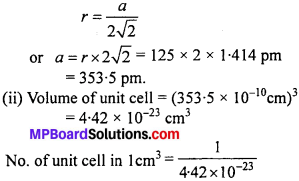Question 25.
If NaCI is doped with 10-3 mol % of SrCl2, what is the concentration of cation vacancies?
Solution:
Introduction of one Sr2+ introduces one cation vacancy because Sr2+ replaces two Na+ ions.
∴ Introduction of 10-3 moles of SrCl2 per 100 moles of NaCl would introduce 10-3 mole cation vacancies.
∴ Number of vacancies per mole of NaCl = $$\frac{10^{-3}}{100}$$ = 10-5 mole = 10-5 × 6.022 × 1023 = 6.02 × 1018 vacancies.

Question 26.
Explain the following with suitable examples :
(i) Ferromagnetism
(ii) Paramagnetism
(iii) Ferrimagnetism
(iv) Anti-ferromagnetism
(v) 12-16 and 13-15 group compounds.
On the basis of magnetic properties solids are classified into the following categories :
(i) Ferromagnetic: A substance that shows unusually large paramagnetism and shows permanent magnetism even in absence of a magnetic field is called ferromagnetic.
Examples : Fe, Co, Ni, CrO2, Fe3O4, alnico (alloy of Al, Ni, Co, Fe and Cu)
A ferromagnetic substance if once magnetised remains magnetised permanently. Ferromagnetism arises due to the spontaneous alignment of magnetic moments (due to unpaired electrons) in the same direction.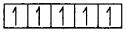(ii) Paramagnetic: A substance which is attracted by a magnetic field is called para-magnetic. Paramagnetism arises due to the presence of permanent dipoles due to unpaired electrons in atoms, ions, or molecules.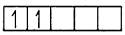Example: Cu+2, Fe3+, TiO, CuO, O2 etc.
(iii) Ferrimagnetic: A substance which shows a fairly good paramagnetic character is called ferrimagnetic.
In ferrimagnetic substances, the alignment of magnetic moments in opposite directions are not equal. As a result, the substance attains net magnetic moments, e.g. Fe3O4 is a ferrimagnetic substance which on heating upto 850 K becomes paramagnetic.(iv) Antiferromagnetic: A substance which shows much-reduced paramagnetism than expected is called antiferromagnetic.
In antiferromagnetic substances, the alignment of magnetic moments is equal and in opposite directions. Hence, the net magnetic moment is zero.
Examples: V2O3,Cr2O3,MnO,Mn2O3,MnO2,FeO,Fe2O3,CoO, Co3O4,NiO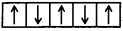(v) 12-16 and 13-15 group compounds: Various types of compounds are formed by the mixture of elements of group 13 and 15 and group 12 and 16 whose average valency is like Ge and Si is 4. Of these specific compounds of group 13-15 are InSb, AlP and GaAs. Gallium arsenious are accelerated sensitive semiconductors. Semiconductors brought a revolutionary change in the manufacture of devices. ZnS, CdS, CdSe, and HgTe are examples of compounds of group 12-16. Bonds of these compounds are not totally covalent and their ionic properties depend on the electronegativity of both the elements present.### The Solid State Other Important Questions and Answers

The Solid State Objective Type Questions

Question 1.

Question 1.
Due to Frenkel defect, the density of ionic solids :
(a) Decreases
(b) Increases
(c) Does not change
(d) It changes.

Question 2.
In CsCl each Cl is surrounded by how many Cs :
(a) 8
(b) 6
(c) 4
(d) 2.

Question 3.
Frenkel defect is not shown by :
(a) AgBr
(b)AgCl
(c) KBr
(d) ZnS.

Question 4.
In NaCl crystal number of oppositely charged ions situated at an equal distance are:
(a) 8
(b) 6
(c) 4
(d) 2.

Question 5.
The best conductor of electricity is:
(a) Diamond
(b) Graphite
(c) Silicon
(d) Carbon (Non-crystalline).

Question 6.
Which type of point defect is found in NaCI crystal or KCl crystal:
(a) Frenkel defect
(b) Schottky defect
(c) Lattice defect
(d) Impurity defect.

Question 7.
How many space lattices (Bravais lattice) can be obtained from various crystal systems:
(a) 7
(b) 14
(c) 32
(d) 230.

Question 8.
Diamond is a:
(a) H-bond solid
(b) Ionic solid
(c) Covalent solid
(d) Glass.

Question 9.
The Co-ordination number of Ca2+ ions in fluoride structure is :
(a) 4
(b) 6
(c) 8
(d) 3.

Question 10.
8 : 8 Co-ordination number is found in which compound :
(a) MgO
(b) Al2O3
(C) CsCl
(d) All of these.

Question 11.
The co-ordination number of body-centered cubic cell is:
(a) 8
(b) 12
(c) 6
(d)4.

Question 12.
The density of unit cell is :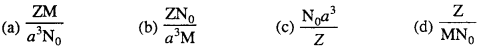Question 13.
The number of tetrahedral voids in the unit cell of cubic close packing :
(a) 4
(b) 8
(c) 6
(d) 2.

Question 14.
Intra-ionic distance of CsCl will be :
(a) a
(b) $$\frac { a }{ 2 }$$
(c) $$\frac{\sqrt{3}}{2} a$$
(d) $$\frac{2 a}{\sqrt{3}}$$

Question 15.
The number of atoms in the body-centered cubic unit cell is:
(a) 1
(b) 2
(c) 3
(d) 4

Question 16.
Which of the following is Bragg equation :
(a) nλ = 2Φ sin θ
(b) nλ = 2d sin θ
(c) nλ = sin θ
(d) $$n \frac{\theta}{2}=\frac{d}{2} \sin \theta$$

Question 17.
Constituents of the covalent crystal are:
(a) Atom
(b) Molecule
(c) Ion
(d) All of these.

Question 18.
Number of Na atom present in the unit cell of NaCl crystal is :
(a) 1
(b) 2
(c) 3
(d) 4.

Question 19.
What type of magnetic substance are Fe, Co, Ni:
(a) Paramagnetic
(b) Ferromagnetic
(c) Diamagnetic
(d) Antiferromagnetic.

Question 20.
The correct example of Frenkel defect is :
(a) NaCI
(b) CsCl
(c) KCl
(d) AgCI.

Question 21.
Dry ice (solid CO2) is a/an :
(a) Ionic crystal
(b) Covalent crystal
(c) Molecular crystal
(d) Metallic crystal.

Question 22.
Co-ordination number of Cs in CsCl:
(a) Like Cl i.e., 6
(b) Like Cl i.e., 8
(c) Unlike Cl i.e., 8
(d) Unlike Cl i.e., 6.

Question 23.
Structure of NaCl crystal:
(a) Tetragonal
(b) Cubic
(c) Orthorhombic
(d) Monoclinic.

Question 24.
Each Na+ ion in NaCl crystal is surrounded by :
(a) Three Cl ions
(b) Eight Cl ions
(c) Four Cl ions
(d) Six Cl ions.

Question 25.
For increasing of electro-conductivity in a solid crystal, mixing of impurities is known as:
(a) Schottky defect
(b) Frenkel defect
(c) Doping
(d) Electronic defect

Question 26.
Which type of lattice is found in KCl crystal:
(a) Face centred cubic
(b) Body centred cubic
(c) Simple cubic
(d) Simple tetragonal.

Question 27.
Number of atoms in a body-centered cubic unit cell of a monoatomic substance is:
(a) 1
(b) 2
(c) 3
(d) 4.

Question 28.
Radius ratio limit for tetrahedral symmetry is :
(a) 0.155
(b) 0.414
(c) 0.732
(d) 0.225.

Question 29.
The defect produced due to a cation and an anion vacancy in a crystal lattice is known as:
(a) Schottky defect
(b) Frenkel defect
(c) Crystal defect
(d) Ionic defect.

Question 30.
If co-ordination number of Cs+ is 8 in CsCl then co-ordination number of Cl ion is :
(a) 8
(b) 4
(c) 6
(d) 12.

1. (c), 2. (a), 3. (c), 4. (b), 5. (b), 6. (b), 7. (b), 8. (c), 9. (c), 10. (c), 11. (a), 12. (a), 13. (b), 14. (c), 15. (b), 16. (b), 17. (a), 18. (d),’ 19. (b), 20. (d), 21. (c), 22. (b), 23. (b), 24. (d), 25. (c), 26. (a), 27. (b), 28. (d), 29 (a), 30. (a).

Question 2.
Fill in the blanks :

1. The defect produced due to the removal of a cation and an anion from a crystal lattice is called ………………..
2. If in a crystal lattice a cation leaves its lattice site and occupies a space in the interstitial site then the defect is called ……………….
3. The cause of electric conduction of NaCl in its molten state is its ………………..
4. Due to ……………….. defect the density of crystal decreases.
5. Total ……………….. types of crystal system are there.
6. ……………….. proposed the concept of the atom for the first time.
7. The ratio of the cation and anion present in a crystal is known as ………………..
8. The process of adding a small amount of impurities in an element or compound is called ………………..
9. Total 14 types of unit cells are there which are known as ………………..
10.  In NaCl crystal structure, the coordination number of both Na+ and Cl ion is ………………..
11. ……………….. defect is found in ZnS and AgCl crystal.
12. Due to the Schottky defect, the density of crystal ………………..
13. In metallic solids, conductivity is due to the presence of …………..
14. Point defects are found in ………….. crystals. Substances which are attracted in the magnetic field are called …………..
15. For a unit cell, if r = $$\frac{a}{\sqrt{8}}$$ then it will be ………….. type of unit cell.
16. The conductivity of semiconductor ………….. on increasing temperature.

1. Schottky defect
2. Frenkel defect
3. Free ions
4. Schottky
5. Seven
8. Doping
9. Bravais lattice
10. Six
11. Frenkel
12. Decreases
13. Free electron
14. Ionic
15. Paramagnetic substance
16. fcc
17. Increases.

Question 3.
Match the following1. (b)
2. (d)
3. (c)
4. (a)1. (c)
2. (d)
3. (a)
4. (b).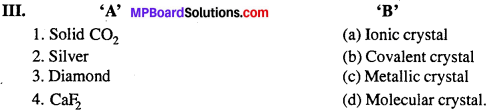1. (d)
2. (c)
3. (b)
4. (a).

Question 4.

1. Give two examples of metallic crystals.
2. Give two examples of covalent crystals.
3. Give two examples of ionic crystal.
4. What is the co-ordination number of F in CaF2?
5. What type of crystal is SiC ?
6. What is the value of the co-ordination number of hexagonal close packing structures?
7. Write the formula of radius ratio.
8. What is the type of structure of NaCl crystal?
9. Give an example of body centered cubic cell.
10. Give an example of a compound which has both Schottky and Frenkel type of defect.
11. Give two examples of amorphous or non-crystalline solid.
12. Write Bragg equation.
13. What is effect on the density of a substance or crystal due to the Schottky defect?
14. State the co-ordination number of CsCl and NaCl.
15. Write the formulae of two superconductors’ substances.
16. Give an example of Frenkel defect.
17. Give an example of a superconductor.
18. The radius ratio of the tetrahedral void is.

1. Copper, Nickel
2. Diamond, Graphite
3. NaCl, NaNO3
4. 4
5. Covalent
6. 12
7.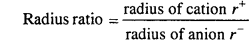8. Cubic
9. CsCl
10. AgBr
11. Glass,plastic,
12. nλ = 2d sin θ
13. Due to schottky defect, density of substance decreases
14. Co-ordination number of CsCl = 8 : 8, Co-ordination number of NaCl = 6:6
15. (i) λBa3- Cu2O7, (ii) Bi2Ca2Sr2Cu3O10
16. AgCl
17. Ba0.7K0.3BIO3
18. 0.225.The Solid State Very Short Answer Type Questions

Question 1.
What are crystalline solids? Crystalline solids are of how many types?
Solids in which the constituent particles like atoms, molecules, or ions are in a definite order, with a definite geometry are known as crystalline solids.

Crystalline solids are of four types:

1. Ionic crystal
2. Covalent crystal
3. Molecular crystal
4. Metallic crystal.

Question 2.
Write the formula of density of unit cell.Question 3.
What is crystal lattice?
Geometry of a crystal in which unit cells are arranged in a definite order and form a crystal-like shape of the unit cell is known as crystal lattice.

Question 4.
What is a unit cell?
The smallest unit formed by the arrangement of constituent particles atoms, ions, or molecules of a crystal in an ordered form is known as the unit cell of the crystal.

Question 5.
Give two-two examples of each of the following :

1. Diamagnetic substance
2. Paramagnetic substance
3. Ferromagnetic substance
4. Antiferromagnetic substance
5. Ferrimagnetism.

1. Diamagnetic substance – TiO2, NaCl
2. Paramagnetic substance – Cu+2, Fe+3
3. Ferromagnetic substance – Fe, Co
4. Antiferromagnetic substance – MnO2, MnO
5. Ferrimagnetism – Fe3O4, Ferrite.

Question 6.
Write the structure and coordination number of the following:
(1) CsCl
(2) NaCl
(3) Zn.
(1) CsCl – Structure : Cubic, Co-ordination number : 8.
(2) NaCl – Structure: Octahedral, Co-ordination number : 6.
(3) Zn – Structure : Tetrahedral, Co-ordination number : 4.

Question 7.
What makes a glass different from a solid such as quartz? Under what conditions could quartz be converted into glass?
Glass is an amorphous solid in which constituent particles are orderly arranged in a short-range order. Quartz is a crystalline form of silica in which SiO4 units are orderly arranged in a long-range order.
Quartz can be converted into glass by melting the glass and then cooling it rapidly.

Question 8.
Which are the seven fundamental crystal systems on the basis of crystal geometry?
Seven types of crystal system are following:

1. Cubic
2. Tetragonal
3. Orthorhombic
4. Monoclinic
5. Hexagonal
6. Rhombohedral
7. Triclinic.

Question 9.
Write the names of different type of cubic system,
Cubic system is of three types :

1. Simple cubic (see)
2. Body centred cubic (bcc)
3. Face centred cubic (fcc).

Question 10.
What is the co-ordination number of Na+ and Cl in the structure of NaCl?
In NaCl structure each Na+ is surrounded by 6 Cl and each Cl is surrounded by 6 Na+ ions.
Thus, Co-ordination number of Na+ = 6
Co-ordination number of Cl = 6.

Question 11.
What is co-ordination number ? What is the effect of temperature and pressure on co-ordination numbers?
The number of neighbouring ions around the constituent particles of a crystal lattice is known as its co-ordination number. At high-pressure co-ordination number increases and at low pressure it decreases.

Question 12.
What information is obtained by X-ray diffraction study of crystals?
By X-ray diffraction study of crystals, spacing between crystal planes of constituents particles of crystals is known.

Question 13.
What type of solids are electrical conductors : metallic or ductile ?
Metallic solids.

Question 14.
Give the significance of a ‘lattice point’.
Each lattice point represents one constituent particle of the solid. The constituent particle may be an atom, a molecule (group of atom) or an ion.

Question 15.
Refractive index of a solid is observed to have the same value along all directions. Comment on the nature of this solid. Would it show cleavage property?
As the solid has same value of refractive index along all directions, this means that it is isotropic and hence amorphous. Being an amorphous solid, it would not show a clean cleavage when cut with a knife. Instead it would break into pieces with irregular surfaces.The Solid State Short Answer Type Questions

Question 1.
What is Schottky defect ?
This type of defect is found in crystals in which both the cation and anion leave their normal lattice site and make their place vacant. In this defect, density decreases but r electrical neutrality is maintained. Like NaCl, CsCl etc.

Question 2.
What is radius ratio of ions ?
Ratio of radii of cation and anion in any crystal is called radius ratio.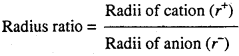For example, in NaCl, ionic radii of Na+ and Cl- ions are 95 pm and 181 pm respectively.
Radius ratio in NaCl = $$\frac { 95 }{ 181 }$$ = 0.52
Due to presence of other forces, in the crystal the observed value of radius ratio is less as in NaCl crystal it is 0414.

Question 3.
Describe briefly the structure of CsCl.
It is AB type ionic crystal with body centred cubic structure. In this Cs+ ion in centre of cube and Cl ions at comers of cube (or vice versa). Co-ordination number of caesium chloride is 8 : 8 and radius ratio of Cs+ and Cl ions is 0.732.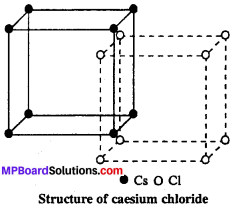In the unit cell of CsCl one Cs+ ion and one Cl ion are present.
Cs+ = 8 (at Centre) × 1 = 1
Cl = 8 (at corners) × $$\frac { 1 }{ 8 }$$ = 1

Question 4.
Calculate the density of unit cell.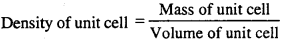If number of particles (atom, molecule, ions) of unit cell is Z and mass of each particle is m, then
Mass of unit cell = m × Z …….(1)
If molar mass of the substance is M, then mass of each particle :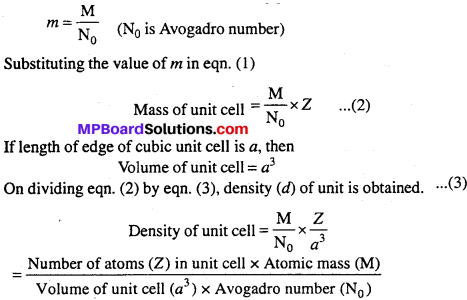Question 5.
Why is glass considered as super cooled liquid ?
Glass is an amorphous solid. Like liquids it has tendency to flow, though very slowly. The proof of this fact is that the glass panes in the windows or doors of old buildings are invariably found to be slightly thicker at the bottom that at the top.

Question 6.
Ionic solids conduct electricity in molten state but not in solid state. Explain.
In the molten state, ionic solids dissociate to give free ions and hence can conduct electricity. However in the solid state, as the ions are not free but remain held together by strong electrostatic forces of attraction, they cannot conduct electricity in the solid state.

Question 7.
What type of defect can arise when a solid is heated ? Which physical property is affected by it and in what way ?
When a solid is heated, vacancy defect is produced in the crystal. This is because on heating some atoms or ions leave the lattice site completely, some lattice sites become vacant. As a result of this defect the density of the substance decreases because some atoms ions leave the crystal completely.

Question 8.
A compound consists of A and B exhibits cubic structure. Atoms are arranged at corners of cube while B are at the centre of faces. What will be the formula of the compound?
Atoms situated at corners are 8 A atoms which are shared by 8 cubes. So in the unit cell:
Number of A atom = 8 × $$\frac { 1 }{ 8 }$$ = 1
B atoms are present in centre of 6 faces and each face is shared by two cubes
So number of B atoms = 6 × $$\frac { 1 }{ 2 }$$ = 3
Formula of compound will be AB3.

Question 9.
Prove that in face centred cubic (fee) structure, there are four atoms in a unit cell.
Face Centred Cubic Structure : In this structure, one atom is also situated in each face along with the corners of cube. The atom in each face is shared by two faces.
Thus, number of atom in each unit cell = 8 × $$\frac { 1 }{ 8 }$$ (at 8 comers) + 6 × $$\frac { 1 }{ 2 }$$ (at 6 faces)
= 1 + 3 = 4.

Question 10.
Write Bragg equation.
Bragg equation is as follows : 2d sin θ = nλ.
Where, d = Distance between two consecutive planes in a crystal,
θ = Incident angle of X-rays
n = Simple whole number
λ. = Wavelength of X-rays.
By this distance d between the planes of the crystal is determined.

Question 11.
Name the parameters that characterise a unit cell.
A unit cell is characterised by (i) Its dimensions along the three edges a, b and c. These edges may or may not be mutually perpendicular
(ii) Angles between the edges, a, (between b and c), β (between a and c) and γ (between a and b). Thus, a unit cell is characterised by six parameters a, b, c, α, β and γ.

Question 12.
Window glass of old buildings appear milky. Why?
In day time, glass becomes hot and cools down at night. This way, the process of annealing takes place. Due to annealing in many years, glass develops crystalline property and window glass appear milky.

Question 13.
Common salt sometimes appear yellow instead of being colourless. Why?
In common salt, due to metal excess defect the anion Cl disappears from its lattice site but leaves an electron thereby which the crystal remains electrically neutral. A hole is formed at the vacant space of anion. This hole is known as F-centre. Due to this reason NaCl appear yellow.

Question 14.
With the increase in temperature, electrical conductivity of semiconductors increases. Why?
The energy gap between valence band and conduction band is less. Thus, with the increase in temperature, some electrons from the valence band jump to the conduction band due to which electrical conductivity increases with the increase in temperature.

Question 15.
Which of the following lattices has the highest packing efficiency :
(i) Simple cubic
(ii) Body centered cubic
(iii) Hexagonal close-packed lattice?
Packing efficiency for simple cubic = 52-4%
Body centred cubic = 68%
Hexagonal close packed = 74%
Hence, Hexagonal close-packed (hcp) has the highest packing efficiency.

Question 16.
What type of stoichiometric defect is shown by (i) ZnS (ii) AgBr?
(i) ZnS shows Frenkel defect because its ions have a large difference in size.
(ii) AgBr shows both Frenkel and Schottky defects.

Question 17.
Explain how many portions of an atom located at (i) Corner and (ii) the Body center of a cubic unit cell is part of its neighbouring unit cell?
(i) An atom at the corner is standing by eight adjacent unit cells. Hence, portion of the atom at the corner not belongs to one unit cell = $$\frac { 1 }{ 8 }$$
(ii) The atom at the body centre of a cubic unit cell is not stand by another unit cell. Hence, it belongs fully to the unit cell.

Question 18.
How can you determine the atomic mass of an unknown metal if you know its density and the dimension of its unit cell? Explain.
Let d – Density of the unit cell and volume of the unit cell = a3 (in case of the cubic crystal)
Mass of an atom present in the unit cellQuestion 19.
The stability of a crystal is reflected in the magnitude of its melting point. Comment. Collect melting point of solid water, ethyl alcohol, diethyl ether, and methane from a data book. What can you say about the intermolecular forces between these molecules?
Higher the melting point, the greater are the forces holding the constituent particles together and hence greater is the stability.
Melting points of the substance are given below: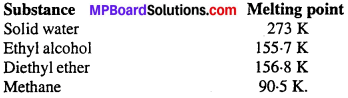Question 20.
A group 14 element is to be converted into an n-type semiconductor by doping it with a suitable impurity. To which group should this impurity belong?
n-type semiconductor means conduction due to the presence of excess negatively charged electrons. Hence, to convert group 14 element into the n-type semiconductor, it should be doped with group 15 element.

Question 1.
Give the difference between crystalline solid and amorphous solid.
Differences between Crystalline solid and Amorphous solid:

Crystalline solid:

1. Structure of crystalline solid are of definite geometrical shape.
2. In the internal structure, particle are arranged systematically.
3. Crystalline solids are solids in real sense.
4. Melting points of these compounds are sharp and definite.
5. These exhibit anisotropy.
6. Cooling curve are not continuous.
7. Crystalline solids possess less energy.

Amorphous solid

1. No any definite geometrical shape in the structure.
2. No any definite arrangement of particles in the internal structure. Amorphous solids are supercooled liquids.
3. Melting point of these compounds are not definite and sharp.
4. These exhibit isotropy.
5. Cooling curves are continuous.
6. Amorphous solids possess higher energy.

Question 2.
Distinguish between :
(i) Hexagonal and monoclinic unit cell
(ii) Face centred and end centred unit cell.
(i) For hexagonal unit cell a = b ≠ c, α = β = 90°, γ = 120°
For monoclinic unit cell a ≠ b ≠ c, α = γ= 90°, β = 90°
(ii) A face-centered unit cell has one constituent particle present at the centre of each face in addition to the particles present at the comers.
An end centred unit cell has one constituent particle each at the centre of any two opposite faces in addition to the particles present at the corners.

Question 3.
Give differences between Schottky and Frenkel defect.
Differences between Schottky and Frenkel defect:

Schottky Defect:

1. Cation and anion completely leave their lattice sites.
2. Density of the crystal decreases by this defect.
3. This defect generally occur in ionic compounds with high co-ordination number where size cation and anion are nearly same.
4. No effect on dielectric constant.

Frenkel Defect:

1. Cation leave its normal lattice site and occupies the intestitial site.
2. No effect on the density of the crystal.
3. It is found in ionic compounds with low co-ordination number and where size of cation and anion differ largely.
4. Magnitude of dielectric constant increase.

Question 4.
What do you understand by imperfections in crystals? What are the rea¬sons for imperfections?
It is generally supposed that arrangement of constituent particles in crystal struc¬ture is completely regular, but actually it is very hard to get a such complete ideal crystal. Crystal structures have many imperfections or defects. Reasons for these imperfections are following:
(i) Temperature: Crystal which have no imperfections or defects are known as ideal crystal. However such crystals exist only at absolute zero temperature because the energy of crystals at 0 K is minimum. At any temperature above 0 K, there are crystals which have some departure from complete order arrangement.

(ii) Presence of impurities: Sometimes the presence of impurities causes disorder in regular arrangement of crystals which is responsible for imperfections and defects.

Question 5.
State the importance of radius ratio in crystal structure.
Importance of radius ratio in crystal structure: Cations have tendency to get surrounded by maximum number of anions, hence larger be the size of cation greater will be its co-ordination number. We can understand this by taking examples of NaCl and CsCl. In NaCl, small sized Na+ has co-ordination number 6, while in CsCl large-sized Cs+ ion has co-ordination number 8. Hence, radius ratio is closely related to co-ordination number.

Radius ratio, Co-ordination number and Structural arrangement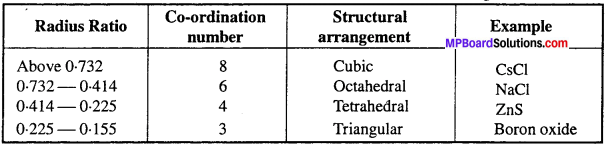Question 6.
Ionic solids, which have anionic vacancies due to metal excess defect develop colour. Explain with the help of suitable example.
Due to anion vacancies basic halides like NaCl, KCl etc., show this type of metal excess defect. Taking the example of NaCl, when its crystals are heated in presence of sodium vapour some chloride ion leave their lattice sites to combine with sodium to form NaCl. For this reaction to occur Na atoms lose electrons to form Na+ ions. The electron has released diffuse into the crystal to occupy the anion vacancies created by Cl ions. The crystal now has excess of sodium. The sites occupied by unpaired electrons are called F- centres. They impart yellow colour to the crystal because they absorb energy from the visible light and get excited.

Question 7.
What type of substances would make better permanent magnets: ferromagnetic or ferrimagnetic? Justify your answer.
Ferromagnetic substances make better permanent magnets. This is because the metal ions of ferromagnetic substances are grouped into small regions called ‘Domains’. Each domain acts as a tiny magnet. These domains are randomly oriented. When the substance is placed in a magnetic field all the domains get oriented in the direction of the magnetic field and a strong magnetic field is produced. This ordering of domains persists even when the external magnetic field is removed. Hence, the ferromagnetic substance becomes a permanent magnet.

Question 8.
How many lattice points are there in one unit cell of each of the following lattice:
(i) Face centered cubic
(ii) Face centered tetragonal
(iii) Body centered.
Solution:
(i) Lattice points in face-centred cubic lattice = 4.
(ii) Face centred tetragonal = 8 (at corners) + 6 (at the face centre) = 14.
However, particles per unit cell
= 8 × $$\frac { 1 }{ 8 }$$ + 6 × $$\frac { 1 }{ 2 }$$
= 1 + 3
= 4.
(iii) Lattice points in body centred cube
= 8 (at comers) + 1 (at the body centre)
= 9
However, particles per unit cell = 8 × $$\frac { 1 }{ 8 }$$ + 1 = 2.### The Solid State Numerical Questions

Question 1.
A compound forms a hexagonal close-packed structure. What is the total number of voids in 0.5 mol of it? How many of these are tetrahedral voids?
Solution:
No. of atoms in the close packing = 0.5 mol
= 0.5 × 6.022 × 1023
= 3.011 × 1023
No. of octahedral voids = No. of atoms in the packing .
= 3.011 × 1023
No. of tetrahedral voids = 2 × No. of atoms in the packing
= 2 × 3.011 × 1023
= 6.022 × 1023
Total No. of voids = 3.011 × 1023 + 6.022 × 1023
= 9.033 × 1023.

Question 2.
A compound is formed by two elements M and N. The elements N forms ccp and atoms of M occupy 1/3rd of the tetrahedral voids. What is the formula of the compound?
Solution:
Suppose the atoms N in the ccp = n
∴ No. of tetrahedral voids = 2n
As 1/3rd of the tetrahedral voids are occupied by atoms M, therefore,
No of atoms M = $$\frac { 2n }{ 3 }$$
∴ Ratio of M : N = $$\frac { 2n }{ 3 }$$ : n
Hence, the formula is M2N3

Question 3.
The structure of a solid AB is like NaCl. Radii of cation A is 100 pm, find out the radii of the anion.
Solution:
For NaCl structure value of radius ratio ($$\frac{r^{+}}{r^{-}}$$) is in between 0.414 – 0.732.
Radius of cation is 100 pm.Question 4.
The radii of A+ and B+ ions are 0.95 Å and 1.81 Å respectively. Find out the co-ordination number of A+.
Or,
The ionic radii of Na+ and Cl are 95 pm and 181 pm respectively.
What will be the co-ordination number of Na+?
Solution:
Radii of Na+ = 95 pm
Radius ratio = $$\frac{r^{+}}{r^{-}}$$ = $$\frac { 95 }{ 181 }$$ = 0.524
Radius ratio is between 0.414 and 0.732. Thus, the co-ordination number of Na+ or A+ will be 6.

Question 5.
Core length of a face centered cubic crystal is 400 pm calculate the density of element Atomic mass of element is 60.
Solution:
Density $$(d)=\frac{Z \times M}{N_{0} \times a^{3}}$$
Where, Z = Number of atoms = 4, in face centred cubic structure.
M = Atomic mass = 60, N0 = Avogadro number (6.023 × 1023)
a = Core length (400 p.m.)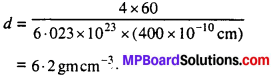Question 6.
Silver crystallises in fee lattice. If edge length of the cell is 4.07 × 10-8 cm and density is 10.5 g cm-3, calculate the atomic mass of silver.
Solution: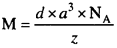Where d = Density of the material
a = Length of the edge of the cell
Z = No. of atoms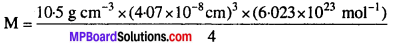∴ Atomic mass of silver = 107.08 g mol-1.

Question 7.
Atomic mass of a face centred cubic (fee) is 60 g mol-1 and the edge of its face is 400 pm. Determine the density of the element
Solution:
Volume of unit cell (a3) = (Length of edge)3
= (400 × 10-12 m)3
= 64 × 10-30 m3
= 64 × 10-30 (102 cm)3
= 64 × 10-24 cm3
Number of atoms in fee unit cell = 4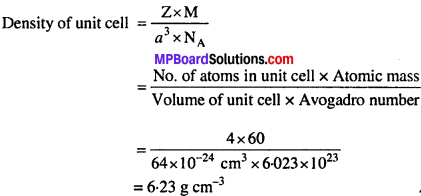Question 8.
Structure of CuCl like ZnS is cubic. If density of CuCl is 3-4 g cm-3, then determine the length of edge of the unit cell.
Solution:
ZnS has a fee structure, thus the same structure will be of CuCl. If length of edge of unit cell is a, number of atoms in fee is Z, molecular mass M and Avogadro number is NA, then,